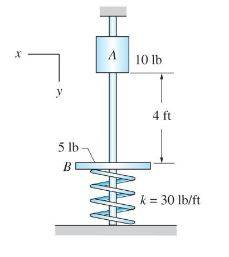# Velocity of a slider immediately before impact

Homework Statement:
1The slider A and the plate B in the figure slide with negligible friction on the vertical guide rod. The plate B is resting on a spring of stiffness k = 30 lb/ft. The slider is released in the position shown and strikes the plate B. The duration of the impact is negligible. Determine the velocity of A immediately before the impact.
Relevant Equations:
Conservation of energy: T1 + K1 = T2 + K2T1 + V1 = T2 + V2
0 + mAgh = 0.5mAV2A1 + 0
40 = (5/32.2)VA2
VA1 = 16.0499 ft/s

Edit: Accidentally Used two notations for Kinetic Energy in the "Useful equations" section. Changed it to correctly notate Kinetic and Potential. This notation is used in R.C. Hibbeler Dynamics 14th Ed.

haruspex
Homework Helper
Gold Member
2020 Award
Problem Statement: 1The slider A and the plate B in the figure slide with negligible friction on the vertical guide rod. The plate B is resting on a spring of stiffness k = 30 lb/ft. The slider is released in the position shown and strikes the plate B. The duration of the impact is negligible. Determine the velocity of A immediately before the impact.
Relevant Equations: Conservation of energy: T1 + K1 = T2 + K2

View attachment 243979
T1 + V1 = T2 + V2
0 + mAgh = 0.5mAV2A1 + 0
40 = (5/32.2)VA2
VA1 = 16.0499 ft/s

Edit: Accidentally Used two notations for Kinetic Energy in the "Useful equations" section. Changed it to correctly notate Kinetic and Potential. This notation is used in R.C. Hibbeler Dynamics 14th Ed.
Your answer is correct, but quotes too many digits for the known precision.

•TaylorHoward21
Your answer is correct, but quotes too many digits for the known precision.
Thank you. I will go back and make sure to include the correct amount of significant figures.